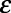## Chapter 8. Fractals

To understand fractals, we have to start with dimensions. The dimension of a space is the number of coordinates we need to specify a point in a space. A number line takes one coordinate, a Euclidean plane takes two, a solid takes three, and so on. See http://en.wikipedia.org/wiki/Dimension.

For simple geometric objects, dimension is defined in terms of scaling behavior; that is, how size depends on length, l. For example, the area of a square is; the exponent, 2, indicates that a square is two-dimensional. Similarly, the volume of a cube is, and a cube is three-dimensional. A line has dimension 1, and if we think of a point as infinitesimally small, it has dimension 0.

Fractal dimension is a more precise and more general extension of this definition. There are several versions; the one I find easiest to understand and apply is the box-counting dimension, which is defined for a set, S, of points in a d-dimensional space. See http://en.wikipedia.org/wiki/Box-counting_dimension.

To compute the box-counting dimension, we divide the space into a grid where the size of each cell is. Then we count , the number of cells that contain at least one element of S. As gets smaller, ...

Get Think Complexity now with the O’Reilly learning platform.

O’Reilly members experience live online training, plus books, videos, and digital content from nearly 200 publishers.